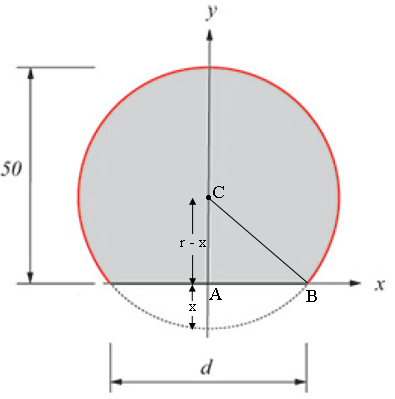SEARCH HOMEMath Central Quandaries & Querieshi there My name is Jennifer and residing in Denmark. I am a student and I wrote to you because i am having trouble in finding out the equation for the circle using (x-a)^2 + (y-b)^2 = r^2.The diameter of this circle is d= 44,514 cm. I have attached a drawing of my problem..thanksHi Jennifer,

I added some lines and labels to the diagram you sent> In particular $C$ is the centre of the circle. Also from your diagram $d$ is not the diameter of the circle but the length of the chord where he circle intersects the X-axis.Triangle $ABC$ is a right triangle so by ythagoras Theorem

$r^2 = (r - x)^2 + \left( \frac{d}{2}\right)^2 .$

From the diagram

$2r - x = 500.$

Solve theis equation for $x,$ substitute into the first equation and solve for $r.$

PennyMath Central is supported by the University of Regina and the Imperial Oil Foundation.